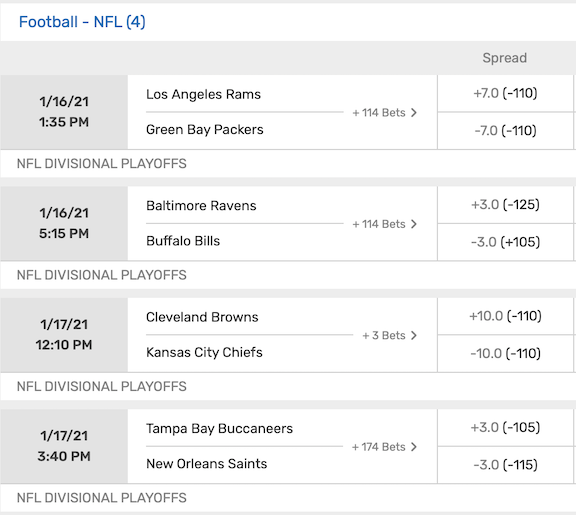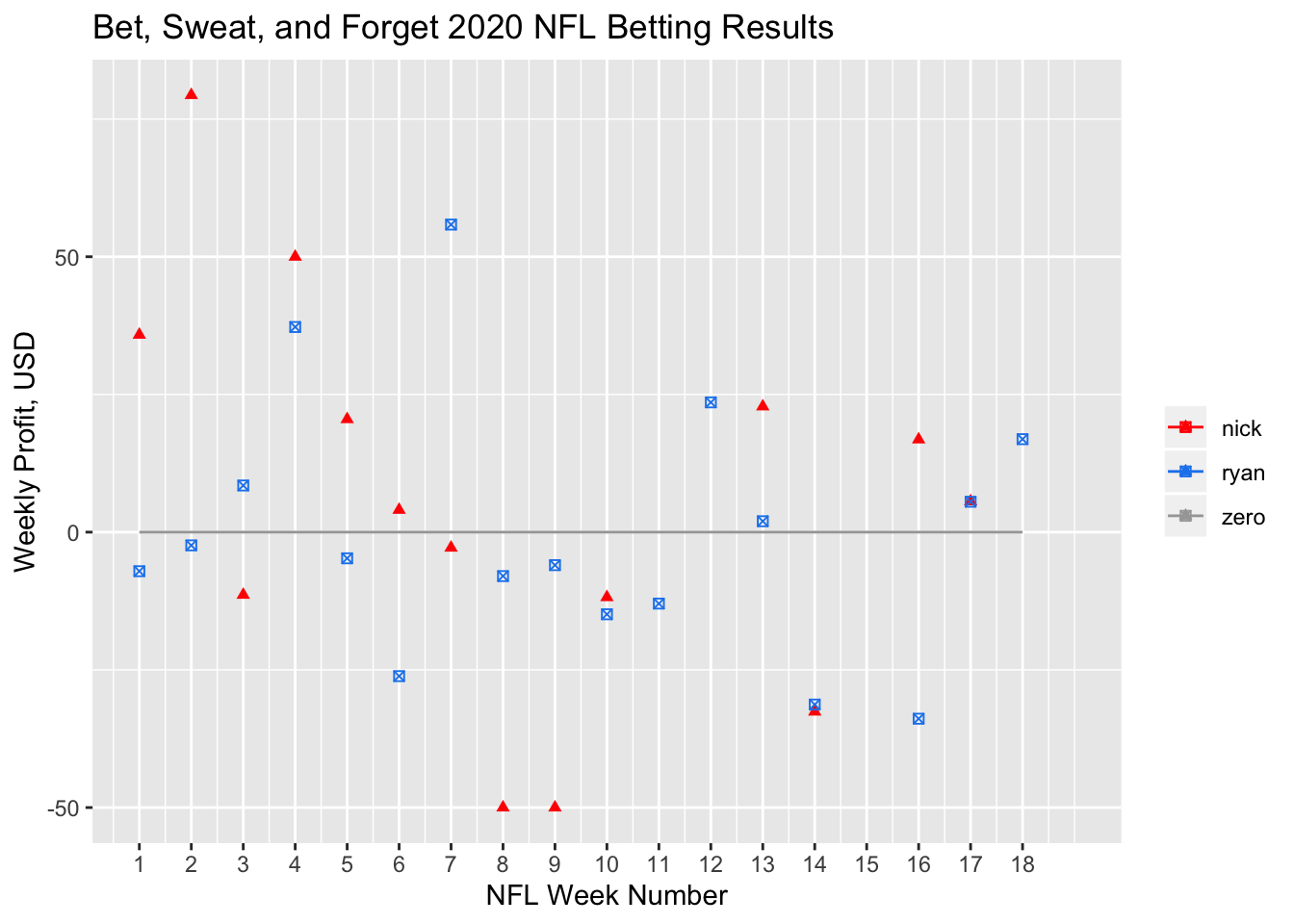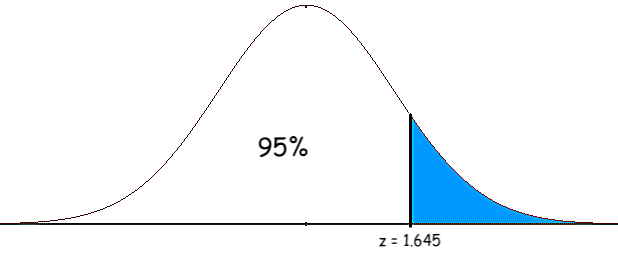# Are Ryan Brill and Nick Miller Better at Sports Betting than a Monkey?

#### Ryan Brill. December 2020.

Each week during the NFL season, Ryan Brill and his buddy Nick Miller make $50 worth of NFL bets on the Bet, Sweat, and Forget Podcast. This begs the following question: Are Ryan and Nick better gamblers than a “monkey throwing darts”, i.e. someone who bets$50 randomly each week?

### Strategy: Conduct a Hypothesis Test

Assume a monkey’s weekly profit is given by the null distribution, which has mean $$\mu_0$$ and variance $$\sigma^2_0$$, which are known constants, to be derived later. Also, suppose Ryan makes \$50 worth of NFL bets in each of $$n$$ weeks. This yields the data $$X_1,...,X_n$$, which are Ryan’s $$n$$ profits for each week of betting. Assume the data $$\{X_i\}_{i=1}^{n}$$ are independently drawn from a distribution with unkown mean $$\mu$$. To determine whether Ryan is better at gambling than a monkey, we shall determine whether Ryan’s weekly profit mean $$\mu$$ is greater than a monkey’s weekly profit mean $$\mu_0$$ with statistical significance. In other words, we conduct a one-tailed hypothesis test: $\begin{cases} \text{null hypothesis: } & \mu = \mu_0 \\ \text{alternative hypothesis: } & \mu > \mu_0. \end{cases}$

Now, let $$\overline{X} = (X_1+\cdots+X_n)/n$$ be the empirical mean of Ryan’s profits. Assuming that Ryan’s profits follow the null distribution, i.e. that Ryan is no better at gambling than a monkey, the Central Limit Theorem then tells us that $Z := \frac{\overline{X}-\mu_0}{\sigma_0/\sqrt{n}} \approx \mathcal{N}(0,1).$

Therefore, we use this value $$Z$$, the z-score, as our test statistic for the hypothesis test.

Then, we compute a confidence interval $$[a,b]$$ such that if Ryan’s weekly profits follow the null distribution, his z-score $$Z$$ should lie inside $$[a,b]$$ with 95% confidence. If Ryan’s observed z-score lies outside the 95% confidence interval, then it is unlikely to have come from the null distribution, and so we reject the null hypothesis, concluding that Ryan is better at gambling than a monkey with 95% statistical significance. Conversely, if Ryan’s observed z-score lies inside the 95% confidence interval, then we do not reject the null hypothesis, and conclude than Ryan is not better at gambling than a monkey with 95% statistical significance.

To summarize, the steps for conducting our hypothesis test are:

1. Compute $$\mu_0$$ and $$\sigma_0$$, the mean and standard deviation of the random variable representing the weekly profit of a sports-betting monkey
2. Compile the data $$X_1,...,X_n$$, Ryan’s $$n$$ weekly profits, and compute the empirical mean $$\overline{X}$$
3. Compute Ryan’s z-score, $$Z = \frac{\overline{X}-\mu_0}{\sigma_0/\sqrt{n}}$$
4. Compute a Confidence Interval $$[a,b]$$
5. Conclude that Ryan is better at gambling than a monkey if and only if $$Z \not\in [a,b]$$

### Computing $$\mu_0$$ and $$\sigma^2_0$$

We model the weekly profit of a sports-betting monkey by a random variable with mean $$\mu_0$$ and variance $$\sigma^2_0$$, which we shall now determine.

Suppose that each week, a monkey places $$\B$$ worth of NFL bets. Suppose that these $$\B$$ are split up into $$N$$ equally sized bets, so each bet is a wager of $$\ B/N$$. Suppose these bets have an average (negative) moneyline of $$-M$$, so $$M\geq 100$$. Now, a “monkey throwing darts” is a common trope referring to an ignorant bettor whose results are determined entirely by chance. Such a monkey has a 50% chance of winning or losing any bet it makes. Therefore, each of a monkey’s $$N$$ weekly profits are given by the random variables $$Y_1,...,Y_N$$, where

$Y_i \overset{iid}{\sim} \begin{cases} -\frac{B}{N} & \text{with probability } 1/2 \text{ (monkey loses its bet}) \\ \frac{B}{N} \cdot \frac{100}{M} & \text{with probability } 1/2 \text{ (monkey wins its bet}). \end{cases}$

The monkey’s total weekly profit is thus given by the random variable $$Y:=Y_1+\cdots+Y_N$$.

We transform these random variables $$\{Y_i\}_{i=1}^{N}$$ into Bernoulli($$1/2$$) random variables $$\{A_i\}_{i=1}^{N}$$ via $A_i := \bigg( Y_i + \frac{B}{N}\bigg)\cdot \frac{NM}{B(100+M)} \overset{iid}{\sim} \text{ Bernoulli}(1/2) = \begin{cases} 0 & \text{with probability } 1/2 \text{ (monkey loses its bet}) \\ 1 & \text{with probability } 1/2 \text{ (monkey wins its bet}). \end{cases}$

Therefore $$A = A_1+\cdots+A_N \sim \text{ Binomial}(N,1/2)$$, so $$\mathbb{E}A = N/2$$ and $$\text{Var}(A) = N/4$$.

Now, inverting the formula for $$A_i$$, we see that $Y_i = A_i \cdot \frac{B(100+M)}{NM} - \frac{B}{N},$

so the monkey’s total weekly profit is given by $Y = Y_1+\cdots+Y_N = A \cdot \frac{B(100+M)}{NM} - B.$

Therefore $\mu_0 := \mathbb{E}Y = \mathbb{E}A \cdot \frac{B(100+M)}{NM} - B = \frac{N}{2} \cdot \frac{B(100+M)}{NM} - B = \frac{B(100-M)}{2M}$

Because $$M$$ is a moneyline, we have $$M\geq 100$$, and so $$\mu_0 \leq 0$$, so a monkey placing sports bets randomly expects to lose money. This makes sense, because if randomly sports betting were profitable, then casinos wouldn’t offer sports bets.

Moreover, $\sigma^2_0 := \text{Var}(Y) = \text{Var}(A) \cdot \frac{B^2(100+M)^2}{N^2M^2} = \frac{N}{4} \cdot \frac{B^2(100+M)^2}{N^2M^2} = \frac{B^2(100+M)^2}{4M^2N}.$

The standard deviation $$\sigma_0$$ of a monkey’s weekly profit is proportional to $$1/N$$, so $$\sigma_0$$ decreases as $$N$$ increases. This makes sense because making $$N=1$$ bet corresponds to “boom or bust” - either you hit the bet and make a lot of money, or you lose the bet and lose a lot of money, which corresponds to high variance. Conversely, making many bets - say, 10 bets - corresponds to lower variance since you have a good chance of hitting 3,4,5,6,or 7 bets, whose corresponding profits are closer to the mean, as oppossed to hitting 0,1,9, or 10 bets.

### Choosing Values for the Constants $$M,B,N$$ in order to Compute $$\mu_0$$ and $$\sigma_0$$

The screenshot below, taken from an online casino, shows the pointspread bets from the 2021 divisional playoff round. This screenshot shows that it is reasonable to take $$M=110$$.Moreoever, during each week of the 2020 NFL season, Ryan Brill made $$\50$$ worth of NFL bets on the Bet, Sweat, and Forget Podcast, so we shall take $$B=50$$.

Thus, using $$B=50$$ and $$M=110$$, we get $$\mu_0 = -2.27$$. In other words, a monkey wagering $$\50$$ profits $$\-2.27$$ on average. This makes sense because our choice of $$-110$$ for the average moneyline indicates that sports betting should be slightly unprofitable.

Furthermore, looking at Ryan Brill and Nick Miller’s betting data from the 2020 Bet, Sweat, and Forget Podcast, we shall take the average number of bets per week to be $$N=4$$, which yields $$\sigma_0 = 23.86$$.

### Computing $$\overline{X}$$ and $$Z$$

Ryan Brill and Nick Millers’ 2020 NFL betting data from the Bet, Sweat, and Forget Podcast are given below:Ryan and Nicks’ empirical means are given by $\overline{X}_{ryan} = 0.12 \qquad \text{and} \qquad \overline{X}_{nick} = 5.45.$

We compute their z-scores via $Z = \frac{\overline{X}-\mu_0}{\sigma_0/\sqrt{n}},$

so Ryan and Nicks’ z-scores are given by $Z_{ryan} = 0.414 \qquad \text{and} \qquad Z_{nick} = 1.21.$

### Confidence Interval

Assume Ryan is no better at gambling than a monkey, i.e. that Ryan’s weekly profit $$X_i$$ follows the monkey’s null distribution with mean $$\mu_0$$ and variance $$\sigma_0^2$$. Then by the Central Limit Theorem, Ryan’s z-score is approximately normal:

$Z = \frac{\overline{X}-\mu_0}{\sigma_0/\sqrt{n}} \approx \mathcal{N}(0,1).$

Now, the 95% quantile for the $$\mathcal{N}(0,1)$$ density function is

round(qnorm(.95),3)
 1.645

which means that 95% of the area under the normal curve is to the left of $$z = 1.645$$:Therefore, under the null distribution, the z-score $$Z$$ lies in the following interval with probability .95:

$[-\infty, 1.645]$

and so this interval is known as a 95% confidence interval.

Both Ryan’s z-score 0.414 and Nick’s z-score 1.21 lie inside the 95% confidence interval $$[-\infty, 1.645]$$, so we fail to reject the null hypothesis for both Ryan and Nick at the 95% significance level. In other words, neither Ryan Brill nor Nick Miller are better at gambling than a monkey, at the 95% statistical significance level.

The following chart illustrates confidence intervals at other significance levels:

Confidence LevelConfidence Interval
85%$$[-\infty, 1.036]$$
90%$$[-\infty, 1.282]$$
95%$$[-\infty, 1.645]$$
97.5%$$[-\infty, 1.96]$$
99%$$[-\infty, 2.326]$$

At the 85% level, Nick’s z-score lies outisde the 85% confidence interval, so we reject the null hypothesis and conclude Nick is better at sports betting than a monkey with 85% statistical significance.